- F_un mathematics - http://cage.ugent.be/~kthas/Fun -

Posted By lievenlb On October 10, 2008 @ 3:03 pm In Connes-Consani2008,undergraduate | 1 Comment

A quick recap of last time . We are trying to make sense of affine varieties over the elusive field with one element, which by Grothendieck’s scheme-philosophy should determine a functorfrom finite Abelian groups to sets, typically giving pretty small sets. Using the F_un mantra thatshould be an algebra overany-variety determines an integral scheme by extension of scalars, as well as a complex variety (by extending further to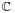). We have already connected the complex variety with the original functor into a gadget that is a couplewhereis the coordinate ring of a complex affine varietyhaving the property that every element ofcan be realized as a-point of. Ringtheoretically this simply means that to every elementthere is an algebra map.

Today we will determine which gadgets determine an integral scheme, and do so uniquely, and call them the sought for affine schemes over.

Let’s begin with our example :being the forgetful functor, that is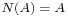for every finite Abelian group, then the complex algebrapartners up to form a gadget because to every element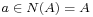there is a natural algebra mapdefined by sending. Clearly, there is an obvious integral form of this complex algebra, namelybut we have already seen that this algebra represents the mini-functorand that the group of unitsof the integral group ringusually is a lot bigger than. So, perhaps there is another less obvious-algebra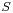doing a much better job at approximating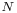? That is, if we can formulate this more precisely…

In general, every-algebradefines a gadgetwith the obvious (that is, extension of scalars) evaluation map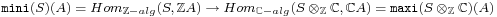Right, so how might one express the fact that the integral affine scheme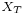with integral algebrais the ‘best’ integral approximation of a gadget. Well, to begin its representing functor should at least contain the information given by, that is,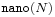is a sub-functor of(meaning that for every finite Abelian groupwe have a natural inclusion). As to the “best”-part, we must express that all other candidates factor through. That is, suppose we have an integral algebraand a morphism of gadgets (as defined last time)then there ought to be-algebra morphismsuch that the above mapfactors through an induced gadget-map.

Fine, but is this definition good enough in our trivial example? In other words, is the “obvious” integral ringthe best integral choice for approximating the forgetful functor? Well, take any finitely generated integral algebra, then saying that there is a morphism of gadgets fromtomeans that there is a-algebra mapsuch that for every finite Abelian groupwe have a commuting diagramHere,is the natural evaluation map defined before sending a group-elementto the algebra map defined byand the vertical map on the right-hand side is extensions by scalars. From this data we must be able to show that the image of the algebra map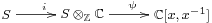is contained in the integral subalgebra. So, take any generatorofthen its imageis a Laurent polynomial of degree say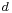(that is,with all coefficients a priori inand we need to talk them into).

Now comes the basic trick : take a cyclic group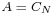of order, then the above commuting diagram applied to the generator of(the evaluation of which is the natural projection map) gives us the commuting diagramwhere the horizontal map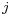is the natural inclusion map. Tracing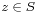along the diagram we see that indeed all coefficients ofhave to be integers! Applying the same argument to the other generators of(possibly for varying values of N) we see that , indeed,and hence thatis the best integral approximation for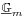.

That is, we have our first example of an affine variety over the field with one element:.

What makes this example work is that the infinite group(of which the complex group-algebra is the algebra) has enough finite Abelian group-quotients. In other words,doesn’t seebut rather its profinite completion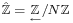… (to be continued when we’ll consider noncommutative-schemes)

In general, an affine-scheme is a gadget with morphism of gadgetsprovided that the integral algebrais the best integral approximation in the sense made explicit before. This rounds up our first attempt to understand the Connes-Consani approach to define geometry overapart from one important omission : we have only considered functors to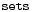, whereas it is crucial in the Connes-Consani paper to consider more generally functors to graded sets. In the final part of this series we’ll explain what that’s all about.